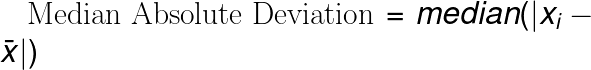# Median Absolute Deviation Calculator

Or input your data as csv

Sharing helps us build more free tools

The median absolute deviation measures the median distance between each point x and the mean of x.

Use this median absolute deviation calculator to calculate the median absolute deviation of a data set.

## Median Absolute Deviation Formula

The median absolute deviation is the median distance between each point and the mean of all points.## What Does the Median Absolute Deviation Tell Us

The median absolute deviation tells us how far we have to go from the mean to reach 50% of points in a data set.

The median absolute deviation is a measure of the spread of data points. Other popular measures of spread are the standard deviation and variance

A large median absolute deviation means that the data is spread.  A small median absolute deviation means that the data is not spread very much.

## What Is the Difference Between the Median Absolute Deviation and the Mean Absolute Deviation

The median absolute deviation is a similar measure to the mean absolute deviation. The mean absolute deviation uses the mean of the deviations to the average while the median absolute deviation uses the median.

Using the median can be more robust to outliers than the mean. This means that the median absolute deviation can be less sensitive to outliers than the mean absolute deviation.

Both the median absolute deviation and the mean absolute deviation have the acronym MAD. This can be kind of confusing.  So when you're using the median absolute deviation or the mean absolute deviation, it can be better to write the full words instead of using the acronym MAD.